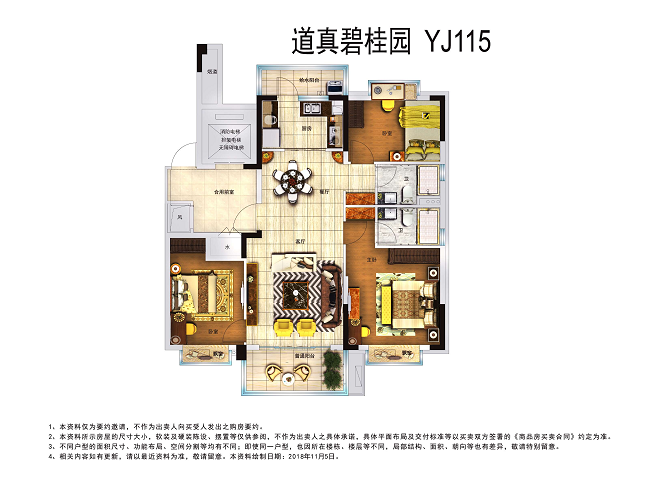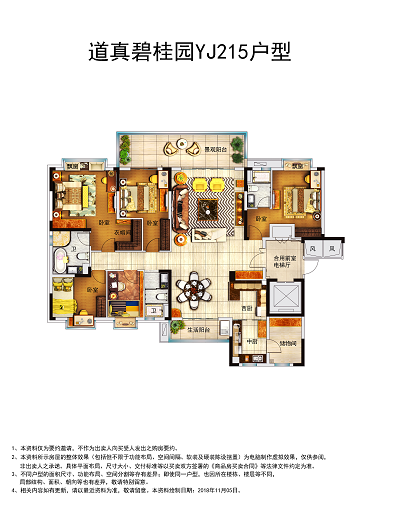## 网站地图## 道真碧桂园

### 遵义

#### 地址

• 资阳
• 本溪
• 北流
• 万载
• 慈溪
• 长春
• 通化
• 西昌
• 丰城
• 阳春
• 鹤山
• 江油
• 辛集
• 辽源
• 衡水
• 黔西南州
• 黔东南州
• 石家庄
• 唐山
• 秦皇岛
• 邯郸
• 邢台
• 保定
• 张家口
• 承德
• 沧州
• 廊坊
• 黄骅
• 迁安
• 怀来
• 洛阳
• 驻马店
• 郑州
• 开封
• 平顶山
• 安阳
• 鹤壁
• 新乡
• 焦作
• 濮阳
• 许昌
• 漯河
• 三门峡
• 南阳
• 商丘
• 信阳
• 周口
• 武汉
• 黄石
• 十堰
• 宜昌
• 襄阳
• 荆州
• 荆门
• 鄂州
• 仙桃
• 潜江
• 黄冈
• 随州
• 咸宁
• 恩施
• 怀化
• 长沙
• 株洲
• 湘潭
• 湘西州
• 张家界
• 益阳
• 郴州
• 衡阳
• 岳阳
• 常德
• 娄底
• 徐州
• 南京
• 无锡
• 镇江
• 泰州
• 淮安
• 舟山
• 黔西南布依族苗族自治州
• 临沂
• 凉山
• 普洱
• 红河
• 锦州
• 葫芦岛
• 东方
• 咸阳
• 丽水
• 定安县
• 吕梁
• 来宾
• 河池
• 宜宾
• 重庆市
• 雅安
• 潮州
• 大理
• 巴中
• 桂林
• 贵港
• 百色
• 柳州
• 贺州
• 儋州
• 福清
• 威海
• 烟台
• 乐山
• 金华
• 永泰
• 潍坊
• 滨州
• 德州
• 临汾
• 日照
• 眉山
• 武威
• 乌鲁木齐
• 保山
• 临沧
• 防城港
• 达州
• 遂宁
• 绵阳
• 淮南
• 宝鸡
• 枣庄
• 桂平
• 景德镇
• 临夏
• 聊城
• 崇左
• 马鞍山
• 安顺
• 北海
• 菏泽
• 铜陵
• 黔南州
• 蚌埠
• 嘉兴
• 淮北
• 都匀
• 南宁
• 兴义
• 南平
• 松溪
• 吉安
• 大同
• 陆丰
• 厦门
• 铜仁
• 银川
• 昭通
• 包头
• 遵化
• 漳州
• 深圳
• 霸州
• 香港
• 合肥
• 抚州
• 琼海
• 定西
• 南昌
• 宿州
• 文昌
• 临高县
• 陵水
• 海口
• 博鳌
• 三亚
• 绥化
• 哈尔滨
• 杭州
• 绍兴
• 宁波
• 温州
• 衢州
• 湖州
• 台州
• 天津
• 沈阳
• 大连
• 通辽
• 兴安盟
• 呼伦贝尔
• 运城
• 晋中
• 太原
• 南通
• 宿迁
• 扬州
• 盐城
• 连云港
• 苏州
• 常州
• 安庆
• 黄山
• 巢湖
• 芜湖
• 池州
• 宣城
• 六安
• 古田
• 无为
• 桐城
• 亳州
• 南陵
• 庐江
• 阜阳
• 滁州
• 孝感
• 天门
• 邵阳
• 永州
• 平果
• 玉林
• 梧州
• 钦州
• 梅州
• 河源
• 云浮
• 东莞
• 汕头
• 中山
• 清远
• 惠州
• 韶关
• 肇庆
• 阳江
• 汕尾
• 茂名
• 江门
• 佛山
• 广州
• 雷州
• 英德
• 湛江
• 揭阳
• 珠海
• 信宜
• 济南
• 淄博
• 青岛
• 泰安
• 济宁
• 马来西亚雪兰莪州
• 马来西亚柔佛州
• 永春
• 南安
• 三明
• 宁德
• 武夷山
• 福鼎
• 莆田
• 建瓯
• 漳浦
• 福安
• 福州
• 泉州
• 龙岩
• 兰州
• 张掖
• 天水
• 白银
• 贵阳
• 仁怀
• 毕节
• 凯里
• 六盘水
• 遵义
• 广元
• 德阳
• 南充
• 泸州
• 攀枝花
• 广安
• 成都
• 宜春
• 萍乡
• 九江
• 赣州
• 上饶
• 曲靖
• 楚雄
• 昆明
• 白城
• 北京
• 澳洲莱德
• 西安
• 渭南
• 上海
• 西宁（商业篇）就目前本项目所处位置的商业配套可谓是“城熟级”，人民法院,县公安局与人民医院就在本项目家门口，涵盖有酒店/餐饮/娱乐/教育/医疗等多功能生活配套，再加上本项目自身配备商业约5500㎡，同样涵盖生活所需的各类配套，如凤凰优选、生活超市、餐饮、咖啡等。

（教育篇）碧桂园自成立以来对教育资源的整合与投入都是每个项目的重中之重，碧桂园选址本区域就是看中周边的教育资源和商业配套。项目周边配有：道真中学/民族小学/民族中学/玉溪小学/玉溪中学等，与本项目距离均在三公里范围之内；业主的适龄子女就读的民族小学、玉溪小学，接送小孩也是便利。

（医疗篇）作为健康的保证，医疗配套也是目前购房因素不可不考虑的，道真县人民医院就在本项目家门口，使得业主生活更加便捷、免除看病难、挂号难的窘境。

1、在实用方面：整个户型设计格局方正，遵循南北通透的居住原理，打造明厨明卫；所有户型均为餐客厅相连，客厅连接观景阳台，在扩大视野感的同时实现对流通风及采光。

2、多空间设计：户型卧室均根据实际设置全景飘窗，尽可能的做大了阳台的面积，增加了阳台的舒适度与实用性，直接拉近与自然美景的距离。

3、干湿分离，动静分区：将面盆区与洗浴区两相分离，有效控制卫生间潮湿，保持室内生活区的洁净清爽；而起居分离，形成休息区与活动区两项独立，考虑到有三代同堂和二胎家庭，将卧室进行分区设置，保障了每位居者的隐私，使生活更加的和谐惬意。

4、想客户所想：在格局布置、尺寸预留上都有考虑，在卧室、厨房、卫生间、走道中预留隐形的收纳空间，即方便业主后期的装修设计，避免了结构改造带来的麻烦，又考虑到因户型收纳空间不足，牺牲了原本属于自己的生活空间，使装修装饰更加整洁，让生活有条不紊。

【全装平墅】建面约117-222㎡